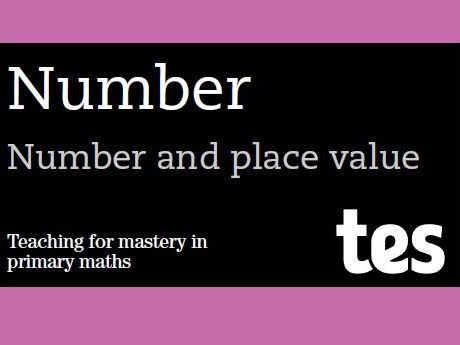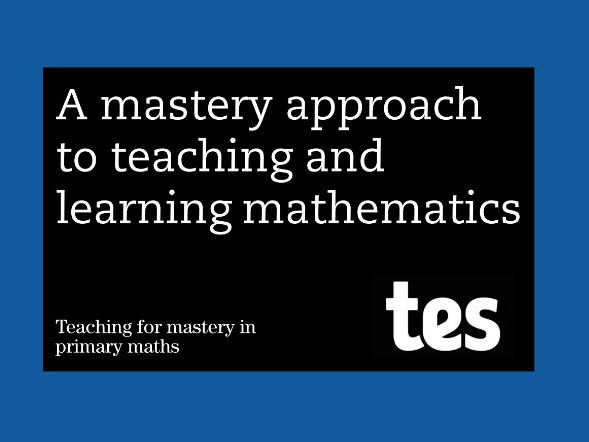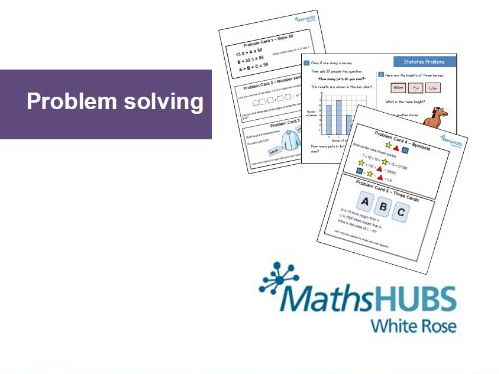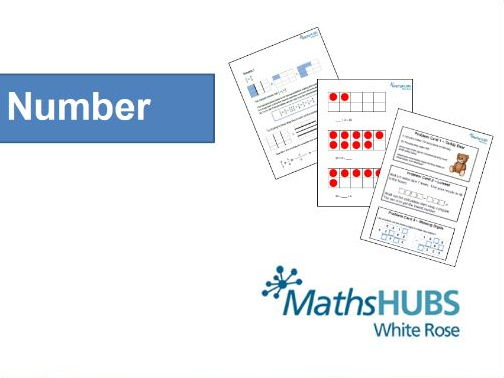# Teaching for mastery in primary maths

## Number: Number and place value

Knowledge of number and place value underpins all other mathematical learning, so the importance of providing pupils with a secure understanding of this topic cannot be understated.

In these chapters, pupils will learn that each digit represents a certain value. As part of this, they will use numbers for counting (1, 2, 3…) and to indicate quantity (2 apples, 3 centimetres). They will also use both numbers and symbols for ordering (first, second, third and 1st, 2nd, 3rd).

Pupils will see how numbers can be partitioned in different ways and then put back together. Ultimately, it is this knowledge that will help them understand addition, subtraction, multiplication and division.

Throughout this learning journey, it is vital that pupils build their understanding of number and place value using varied concrete and pictorial representations. The use of these representations should not just be confined to the early chapters.

### Teaching number and place value

Bring primary pupils up to speed with number and place value, the building blocks of maths education.

### Chapter 1: Numbers up to 10

In this chapter, pupils are introduced to the numbers zero to 10. It is essential that time is invested in this content so that pupils’ knowledge is secure before moving on.

Pupils count forwards and backwards within 10 and up to 10 objects. They begin to compare quantities of objects by using language such as “equal to”, “more than”, “less than”, “fewer”, “most” and “least”. Given a number, they also identify “one more” or “one less”.

Throughout this chapter, pupils read and write numbers to 10 in numerals and words. They ought to experience many different representations of numbers and should represent numbers using objects. For example, they may use cubes or Dienes to feel and see the size of a number and make comparisons. Additionally, they may use ten frames to represent numbers, as well as pictorial representations, such as the number line.

Although many pupils will already seem able to perform much of the above, this apparent fluency should not be mistaken for the required depth of conceptual understanding.

### Chapter 2: Numbers up to 20

In this chapter, pupils are introduced to the numbers 11 to 20. It takes a similar approach to the previous chapter in that pupils continue to practise counting, comparing and ordering numbers.

The key to this chapter, however, is that pupils start to partition numbers above 10 into tens and ones. They use part-whole diagrams to reinforce their understanding and, as before, work with many different concrete and pictorial representations of numbers.

Pupils continue to use language, including “equal to”, “more than”, “less than”, “fewer”, “most” and “least”. They count forwards or backwards to 20, beginning at 0 or 1, or from any given number. They can also identify “one more” and “one less” than a given number.

This chapter lays the essential foundations of place value, as pupils begin to recognise the place value of each digit in a two-digit number (ie, in tens and ones).

### Chapter 3: Numbers up to 40 or 50

In this chapter, pupils extend their representational work to include numbers up to 40 or 50. This helps to reinforce the place value of each digit in a two-digit number (ie, in tens and ones).

Pupils have opportunities to identify and represent numbers using objects, such as counters on a ten frame, cubes, Dienes and pictorial representations, including the number line and part-whole diagrams.

They continue to use “equal to”, “more than”, “less than”, “fewer”, “most” and “least” when talking about number. They count forwards and backwards to 40, beginning with 0 or 1, or from any given number. They can also identify “one more” and “one less” than a given number.

This chapter builds on the essential foundations of place value, in preparation for working with numbers to 100.

### Chapter 4: Numbers up to 100

In this chapter, pupils begin to recognise place value in numbers beyond 40 or 50 by reading, writing, counting and comparing numbers up to 100, supported by objects and pictorial representations.

Pupils count forwards and backwards to and across 100, beginning with any given number including 0 and 1. They also count up to 100 objects. This is not simply a case of chanting the numbers by rote. Rather, pupils gain an understanding of a number, such as 68, by representing it in many different ways. They identify and represent numbers using objects such as Dienes, cubes and pictorial representations, including the number line and part-whole diagrams. They may also use a place value grid to show the value of each digit in a number.

In line with expectations in literacy, pupils read and write numbers to at least 100 in numerals and in words and use mathematical language, such as “equal to”, “more than”, “less than”, “fewer”, “most” and “least”.

### Chapter 5: Two-digit numbers

In this chapter, pupils recognise the place value of each digit in a two-digit number (ie, in tens and ones) and begin to understand zero as a place holder.

This occurs through frequent opportunities to identify, represent and estimate numbers to 100 using different representations, including the number line.

Pupils partition numbers in different ways (eg, 23 = 20 + 3 and 23 = 10 + 13). They show this using part-whole diagrams or using Dienes, counters, cubes or place value grids to support their understanding.

Much of the mathematical content of this chapter involves deepening pupils’ understanding of concepts encountered previously. This might be through the introduction of a greater variety of representations (either pupils moving interchangeably between or selecting their own representations), practising for greater fluency and independence with language and asking and answering increasingly sophisticated mathematical questions.

This chapter also sees the introduction of inequality notation, as pupils compare and order numbers from 0 up to 100 using <, > and = signs.

### Chapter 6: Three-digit numbers

In this chapter, pupils identify, represent and estimate numbers to at least 1,000 (ie, hundreds, tens and ones) using a variety of representations, including those related to measure.

They compare and order numbers up to 1,000 and they apply partitioning related to place value (eg, 146 = 100 + 40 + 6, 146 = 130 + 16). As ever, it is important that concrete and pictorial representations are used throughout.

Many children have been learning to say which number is “one more” or “one less” than a given number since before starting school; first with numbers within 5, then within 10, then within 100 and now within 1,000. This is extended further in this chapter by asking pupils to find 10 and 100 more or less than a given number. This helps pupils deepen their understanding of place value, particularly when working with numbers that move into the next 10 or 100.

### Chapter 7: Four-digit numbers

In this chapter, pupils’ recognition of place value to four-digit numbers (ie, thousands, hundreds, tens and ones) is extended.

As always with work on number and place value, it is important that pupils identify, represent and estimate numbers using different representations. Once again, it is important that concrete and pictorial representations are used throughout.

Pupils learn to round to the nearest 10, 100 or 1,000 and connect estimation and rounding numbers to the use of measuring instruments. In this chapter, pupils are also asked to find 1,000 more or less than a given number in addition to finding 1, 10 and 100 more or less.

### Chapter 8: At least 1 million

In this chapter, pupils extend their understanding of the number system to include larger integers. The skills and concepts build on those from all previous number and place value chapters.

Pupils learn to read and write numbers to at least 1 million. They determine the place value of each digit, using this to order and compare numbers. They count forwards or backwards in steps of powers of 10 and round to the nearest 10, 100, 1,000, 10,000 and 100,000.

### Chapter 9: Positive integers

In this final chapter, pupils work with any sized numbers in a variety of contexts. This is a chance for them to explore real-life situations and solve practical problems within authentic contexts.

Pupils will have solved plenty of real-life problems in previous chapters, but as many very large numbers appear in science and nature, opportunity can now be sought to explore these larger numbers across the curriculum.

All of the work in this chapter reinforces the concepts from previous ones. For example, pupils should still be able to determine the place value of each digit within a number, compare and order numbers, as well as finding 10, 100 and 1,000 more or less than a given number.#### Number and place value: Teaching for mastery booklet

Brought to you by Tes in partnership with experts at Mathematics Mastery and the White Rose Maths Hub, this PDF provides teachers with the progression of learning for the topic, broken down into digestible chapters.

It is an ideal starting point for primary teachers looking to brush up on their subject knowledge, or adopt the mastery approach to maths in their classroom.

Check out the Teaching for mastery site, which contains schemes of work accompanied by quality-assured classroom resources.

Download resources to match this topic in the planning and lesson resources pages of the new primary maths section.#### Teacher's guide: Teaching for mastery booklet

Brought to you by Tes in partnership with experts at Mathematics Mastery and the White Rose Maths Hub, this PDF provides teachers with the progression of learning for the topic, broken down into digestible chapters.

It is an ideal starting point for primary teachers looking to brush up on their subject knowledge, or adopt the mastery approach to maths in their classroom.

Check out the Teaching for mastery site, which contains schemes of work accompanied by quality-assured classroom resources.

Download resources for all primary maths topics in the planning and lesson resources pages of the new primary maths section.

## Approved resources#### Place Value Word Problems

A range of word problems involving place value with 6 digit numbers and up to 2decimal places. Worksheet attached#### Place value powerpoints

Place value powerpoints concerning partitioning numbers, arrow cards, abacus and dienes.#### Place value with Pirate Pete

A powerpoint using a pirate character counting gold coins to help understand place value and link to using base ten materials#### Simple place value investigation

Powerpoint introducing a simple place value investigation for finding all possibilities. Pupils find the answer to the question how many different 4 digit numbers can be made by arranging these 4 digits.#### Place Value Number Detectives

Put a Post-It note on each square so that the children can follow the clues and write their answer on top. Work down each column and write the possible answers on the Post-It notes. They should arrive at the correct number in the final square. A3 resource (Post-It Note sized squares).

## Related resources#### Reasoning - Problem Solving - Money Problems (KS1/2) - March 8th

Today's problems are ones that involve reasoning and problem solving with the numbers. There are two problems for KS1 and two for KS2.

In KS1 problem children have to use a number line to work out the size of the interval and then locate a particular number. Children then have to work out the highest and lowest numbers you can make given three digit cards. Version 2 of the Y2 problems has a slightly less demanding problem Q1 that might be good as a warm up.

At KS2 children also need to find intervals on a number line, but the problem is a little more involved.. The second problem should be tacked systematically.

Every day in March the White Rose Maths Hub is aiming to put out a question of the day.

Tweet us @WRMathsHub a picture of your children's answers for a chance to win a prize.#### Multiplying and Dividing by 10, 100, 1000

This is a worksheet on multiplying and dividing whole numbers (and some decimals) by 10, 100, 1000. Pupils should be encouraged to use place value grids and counters to help them understand what happens.

The worksheets works through some quite basic fluency to more reasoning and problem solving questions that test a deeper understanding of the concept.#### Place Value - 4 digit numbers

This worksheet checks pupils understanding of 4 digit numbers. It is designed to be used more at the end of a sequence of work on place value (4 digit numbers). Pupils should be encouraged to use place value cards and counters to help them understand, reason and explain what they are doing.

The questions start quite fluency based testing students understanding of place value in numbers. They then have to find 10, 100 and 1000 more than given numbers. They then have to order numbers and identify values on a number line.